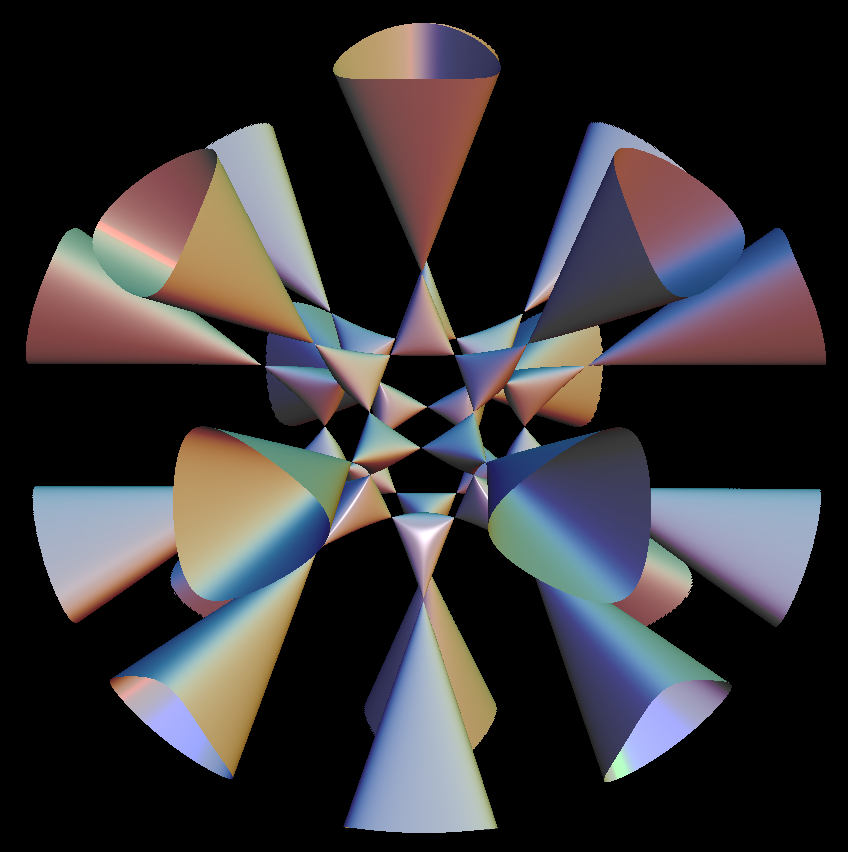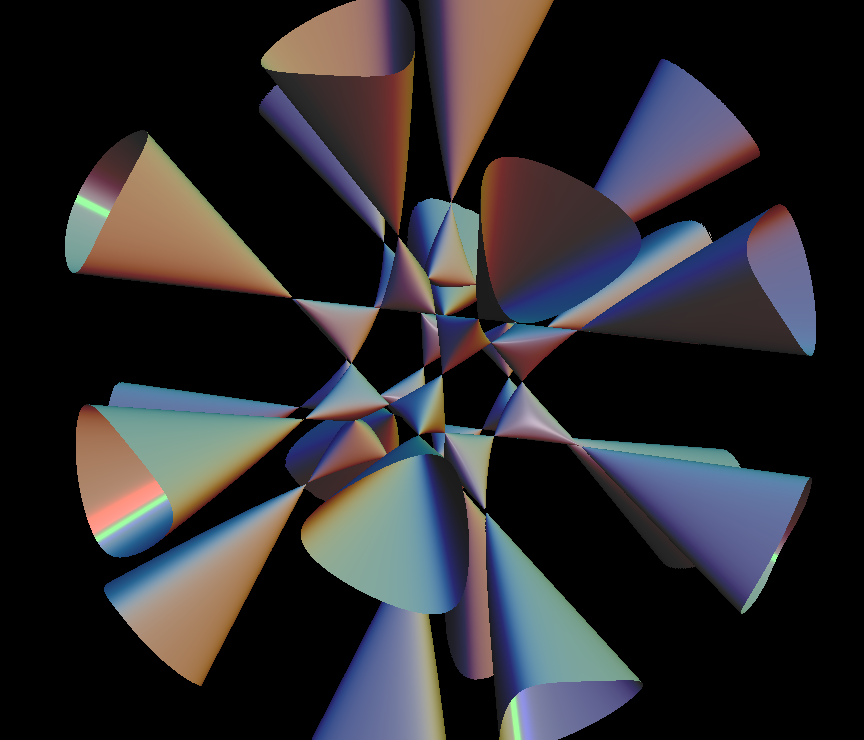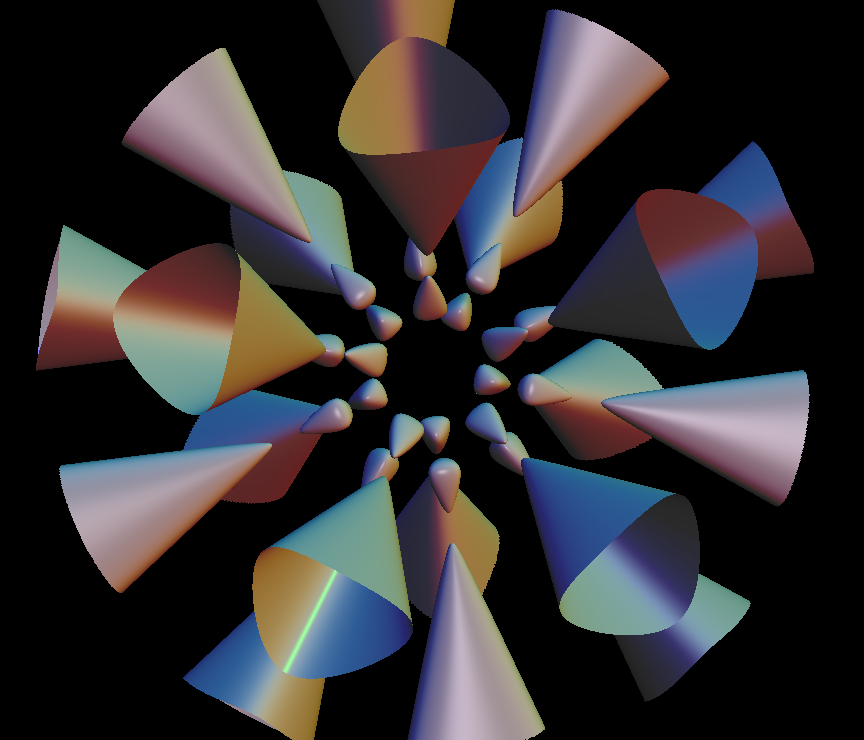# Barth Sexticbarth sexticThis complicated surface is a level surface of a polynomial that has only degree 6. No other degree 6 polynomial with as many conical singularities is known.Observe the beautiful icosahedral symmetry of the level surfaces in this animation. The transition from the separated pieces through the singular level to the connected levels is called a Morse transition.

Morse Theory

This theory says:

If you have on a compact differentiable manifold M a differentiable function

f : M ⟶ R

such that at every critical point p (i.e. grad f(p) = 0 ) the (symmetric) matrix of second partial derivatives has no 0 eigenvalue, then f is called a Morse function.

The topology of the sublevels of f (i.e. f^{-1} ( [min(f), x] ) ) changes only if x is a critical value (i.e. there exists p with f(p) = x and grad f(p) = 0). The way the topology changes, is determined by the index of the matrix of second partial derivatives at the critical point p.

Our animation sequence shows an example of this change of topology.

For more detail, see Morse theory